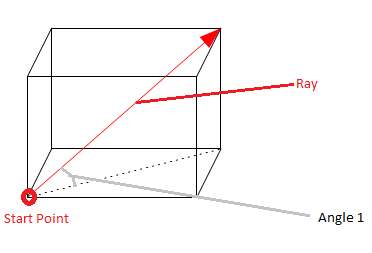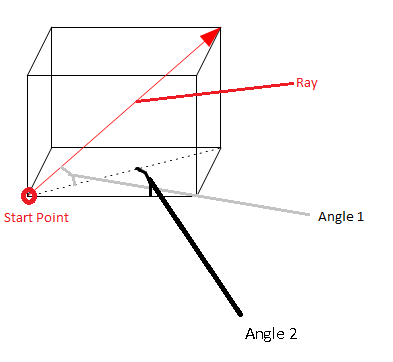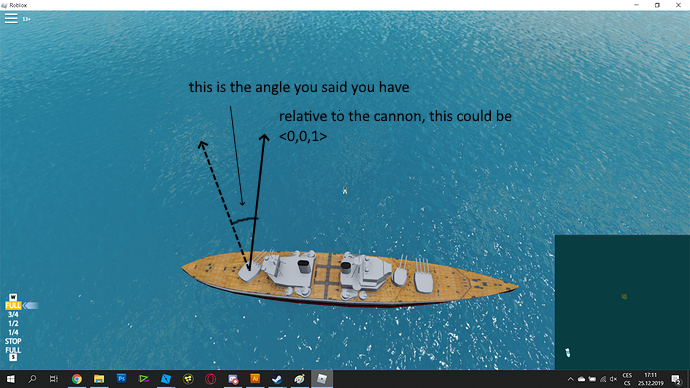# How to create ray from vector3 and angle?

Hi,
I need to create ray, that have start point at vector3, and its direction will be from 2 angles, but i have no idea to do it.

Ehm…Can u please make a drawing? It would make it a lot easier to understand^^

3 Likesand this for both axis (when i rotate it in third axis, it will be same)

1 Like

Okay, so, InverseTransformDirection converts a direction from world space into local space. this doesn’t make much sense. I guess you want it the other way round (local to world).

I still don’t get it. Im sorry. Do you mean something like this?yes, exactly this (you have there only 1 angle, but i need to create it from horizontal and vertical angle, but i think, you just forgot to draw it)

I didn’t forgot to draw. I just didn’t know where to put it. Can u copy my drawing and insert the 2nd angle?I think you need to use Trigonometric functions for that, but I don’t know anymore how to use them. What do you need this for? Maybe there’s another solution for problem.

finding where are canons are aiming

If you want to make a gun this tutorial from Alvinblox might be helpful. Another Option would be the FastCast Plugin:

I have quite more specific problem

I know vertical and horizontal alngle of cannons and i need to know the target of them

Since you have the angles of the cannon, you could use the 2D rotation matrix to find the direction.

If you have one vector where the cannons initially point toward, in order to apply a change of angle, you would use the 2D rotation matrix.
(info on rotation matrix: Rotation matrix - Wikipedia)

For example, if your cannon initially pointed like this:to change the horizontal angle, you could do this:

``````local cannonDir = Vector3.new(0,0,1)
local horizAngle = math.pi/6

local dirAfterApplyingHorizontalAngle = Vector3.new(
cannonDir.X*math.cos(horizAngle) - cannonDir.Y*math.sin(horizAngle)
,0
,cannonDir.X*math.sin(horizAngle) + cannonDir.Y*math.cos(horizAngle)
)
``````

Then, you could use this same logic to change the vertical angle.

Hope this helps!

2 Likes

what is the cannonDir???

It is some direction that your cannon initially points to.

For now, consider just the horizontal angle. In order to have an angle, you have two lines. One of those lines points in the direction of the cannon after you change the angle. The other is the initial direction of the cannon. cannonDir is this initial directionBy the way, as of now, does your cannon rotate (via mouse)?

Ya, the cannon rotate to mouse.Hit

Does it rotate on one plane only?